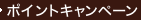•### Regression and Anova : An Integrated Approach Using Sas Software

• ウェブストア価格 ¥22,317（本体¥20,289）
• John Wiley & Sons Inc（2003/05発売）
• 外貨定価 US\$ 133.00
• ウェブストア限定 全点ポイント5倍キャンペーン（6/24～6/28）
• ポイント 1,010pt
• 提携先の海外書籍取次会社に在庫がございます。通常3週間で発送いたします。
重要ご説明事項
1. 納期遅延や、ご入手不能となる場合が若干ございます。
2. 複数冊ご注文の場合、分割発送となる場合がございます。
3. 美品のご指定は承りかねます。
• 【重要：入荷遅延について】
ウクライナ情勢悪化・新型コロナウィルス感染拡大により、洋書・洋古書の入荷が不安定になっています。詳しくはこちらをご確認ください。
海外からのお取り寄せの場合、弊社サイト内で表示している標準的な納期よりもお届けまでに日数がかかる見込みでございます。
申し訳ございませんが、あらかじめご了承くださいますようお願い申し上げます。
• ◆画像の表紙や帯等は実物とは異なる場合があります。
• 製本 Paperback:紙装版/ペーパーバック版／ページ数 592 p.
• 言語 ENG
• 商品コード 9780471469438
• DDC分類 519.536

### Full Description

The information contained in this book has served as the basis for a graduate-level biostatistics class at the University of North Carolina at Chapel Hill. The book focuses in the General Linear Model (GLM) theory, stated in matrix terms, which provides a more compact, clear, and unified presentation of regression of ANOVA than do traditional sums of squares and scalar equations. The book contains a balanced treatment of regression and ANOVA yet is very compact. Reflecting current computational practice, most sums of squares formulas and associated theory, especially in ANOVA, are not included. The text contains almost no proofs, despite the presence of a large number of basic theoretical results. Many numerical examples are provided, and include both the SAS code and equivalent mathematical representation needed to produce the outputs that are presented. All exercises involve only "real" data, collected in the course of scientific research. The book is divided into sections covering the following topics: Basic Theory Multiple Regression Model Building and Evaluation ANOVA ANCOVA

`    Preface.    Examples and Limits of the GLM.    Statement of the Model, Estimation, and    Testing.    Some Distributions for the GLM.    Multiple Regression: General Considerations.    Testing Hypotheses in Multiple Regression.    Correlations.    GLM Assumption Diagnosti`